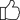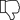Fullerton India Credit Co. Ltd. is Now SMFG India Credit Co. Ltd.

# Apr Vs Apy - What Are The Differences?

Published on Sept 15, 2022Updated on Oct 10, 2023We have to pay interest to the lender for credit and loans. On savings or money accumulated, the bank pays us interest –The concepts of Annual Percentage Rate (APR), and Annual Percentage Yield (APY) are linked to lending and savings respectively.

You’ll see APR and APY terms used in conjunction with credit cards and loans. It is easy to confuse both these terms as both involve interest rates. But what are they, and why are they important? In this guide, we explain all you need to know.

Let us understand each concept in depth.

## What is the Annual Percentage Rate (APR)?

In simple terms, the annual percentage rate is the amount of simple interest rate you’ll be charged by a lender. APR not only includes interest rate but also any additional fees levied such as lending fees, loan origination, processing fees, settlement charges, and more. The entire amount is calculated on a yearly basis and is expressed as a percentage. One can get an estimation of true costs of borrowing from the lender and helps you look past initial rates and fees.

You’ll need to take expected fees and interest on loan into consideration while calculating APR.

The formula for calculating APR is –

APR = ((Fees + Interest / Principal) / n) * 365) * 100
n = the number of days included in the loan term.
Principal = the total amount of loan taken up.
Interest = total interest payable/ paid over the lifetime.

Must Read: Difference Between Reducing Balance and Flat Rate Interest

## What is Annual Percentage Yield (APY)?

The Annual Percentage Yield is an indicator of total money earned by taking compounding interest into consideration. Financial institutions usually use APY to describe an interest rate compounded on savings accounts, investments or FDs.

The formula that is utilized for the calculation of APY is –

APY = (1 + r / n) ^n – 1
r = the period rate.
n = the number of compounding periods.
Now that we have understood these 2 concepts, let us understand what is the difference between annual percentage rate and annual percentage yield.

## APR V/S APY:

1. Rate of Interest –
While APR is used in the context of borrowing, APY generally discusses money earned from investments and deposits.
In the case of APR, the interest rate when lower, is better. It is because APR indicates the money that is owed by you, and lesser money paid works economically for you. On the other hand, the higher the APY, the better it is. Here, it means that if the APY is high, the money you earn on your savings and investments is higher. Thus, it works in your best interest.
2. Calculation –
APR and APY both have a common factor of interest rate but there are additional factors to weigh into which affect their calculations. In APR, interest payable on the loan amount is not the only expense. Other charges like lending fees, settlement costs, processing fees, and other charges are included. In APY, the interest rate is the main criterion but the compounding factor is included thereby giving an accurate idea about the earning potential. In APY, compounding is factored in whereas APR does not include the same.
3. Variation In Rates –
If the interest rate is fixed then the chances of alteration in the same are unlikely. On the other hand, if it is fluctuating it can affect your pocket deeply. Fluctuations in APR and APY can be caused due to market fluctuations.

## Conclusion:

For effective management of your finances, understanding the concept of APR and APY is crucial. The power of compounding needs to be weighed and the difference between the APR and APY rates needs to be evaluated. Comparing rates provided by various lenders helps you understand what will be favourable for you. It is important to be aware of the different rates to make well-informed and wise decisions relating to your finances. To know the APR for your loan, you can use our free APR calculator.

YesNo